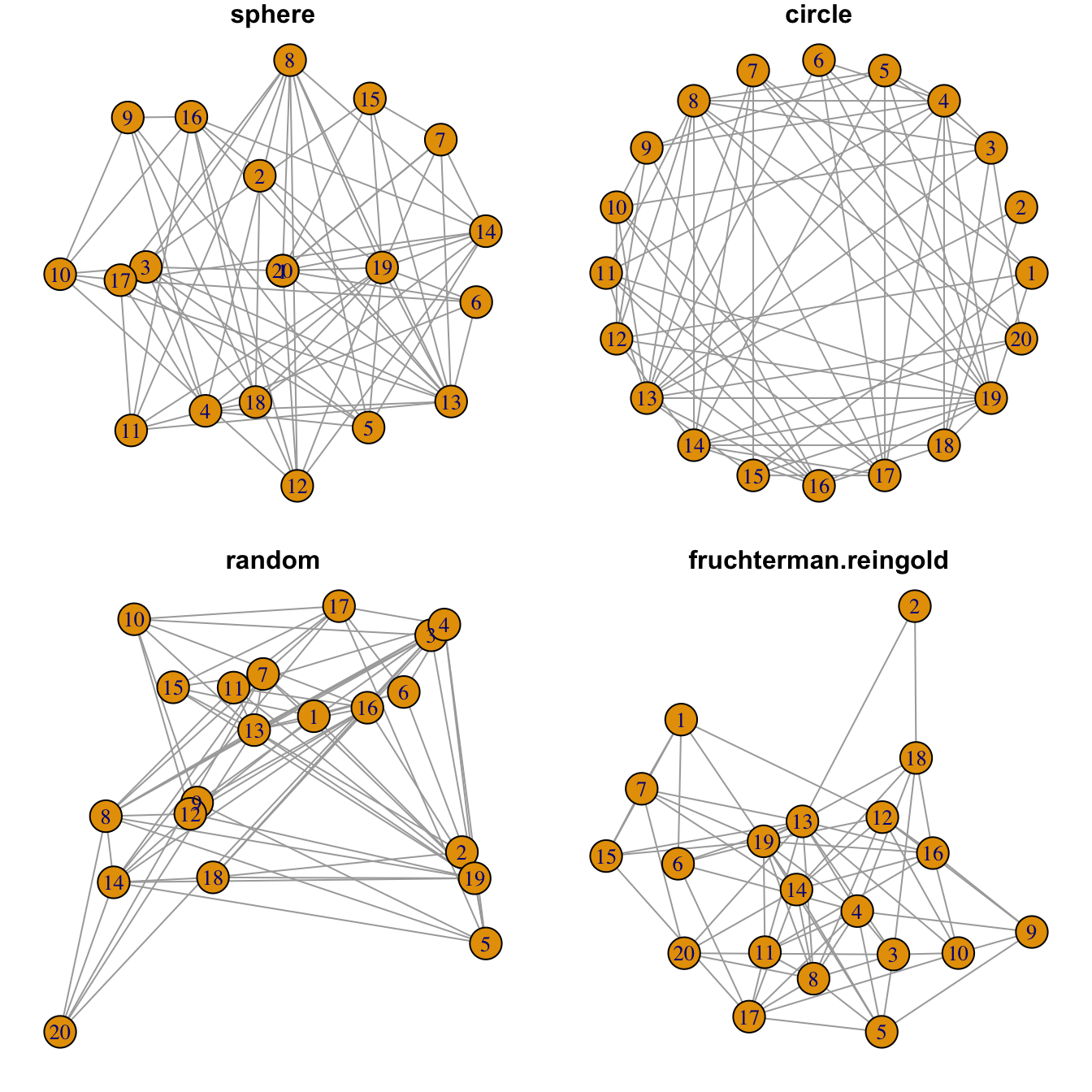# Network graph layouts with R and igraph

This post describes how to apply different layouts to a network diagram using the `igraph` R library. It gives reproducible code showing how to use the offered algorithms.

Network layouts are algorithms that return coordinates for each node in a network. The `igraph` library offers several built-in layouts, and a sample of them is presented here.

Usually, algorithms try to minimize edge crossing and prevent overlap. Edges can have uniform length or not.

Choose your layout using the `layout` argument. Type `help(layout)` to see all the possibilities.

In practice, the `fruchterman reingold` algorythm is often used.``````# library
library(igraph)

# Create data
data <- matrix(sample(0:1, 400, replace=TRUE, prob=c(0.8,0.2)), nrow=20)
network <- graph_from_adjacency_matrix(data , mode='undirected', diag=F )

# When ploting, we can use different layouts:
par(mfrow=c(2,2), mar=c(1,1,1,1))
plot(network, layout=layout.sphere, main="sphere")
plot(network, layout=layout.circle, main="circle")
plot(network, layout=layout.random, main="random")
plot(network, layout=layout.fruchterman.reingold, main="fruchterman.reingold")

# See the complete list with
# help(layout)``````

Related chart types

## Contact

This document is a work by Yan Holtz. Any feedback is highly encouraged. You can fill an issue on Github, drop me a message on Twitter, or send an email pasting yan.holtz.data with gmail.com.